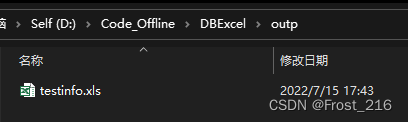### 导入：

#### 1. 读取excel文件(这里可以写死路径，也可以用相对引用路径)

tips: xlrd 这个包最新的 2.0版本不支持读取 xlsx 文件

``````pip install pandas
pip install xlrd==1.2.0
pip install xlwt==1.3.0``````

``````import pandas as pd

# 以下两种方式均可
# 这种是路径写死的方式
# 查看 df
print(df)``````

df 包含了 excel 表中的数据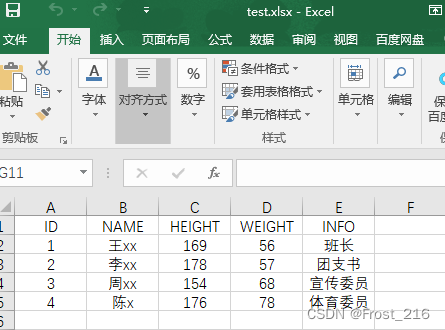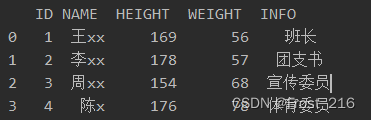``````print("excel总行数为: ", len(df), "\n")

for i in range(len(df)):
print(i)``````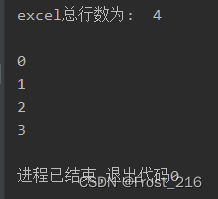#### 2. 创建与SqlServer的连接 (其他数据库也一样，这里用的SqlServer)

``pip install pymssql``

``import pymssql``

``````def conn():
# 后续如果出现乱码 请调整此处的 charset 换成utf-8
connect = pymssql.connect('.', 'sa', '123', 'DBCenter', charset='GBK')
if connect:
print("连接成功")
return connect``````

#### 3. 接下来就通过读取的excel向数据库中插入

``````# 第一种方式
for i in range(len(df)):
id = df['ID'].values[i]
name = df['NAME'].values[i]
height = df['HEIGHT'].values[i]
weight = df['WEIGHT'].values[i]

strsql = f"INSERT INTO testinfo SELECT {id},'{name}',{height},{weight}"
print(strsql)

print('1 ↑\n\n2 ↓')

# 第二种方式
# 通过 iloc 寻找固定行列值
for i in range(len(df)):
id = df.iloc[i]
name = df.iloc[i]
height = df.iloc[i]
weight = df.iloc[i]

strsql = f"INSERT INTO testinfo SELECT {id},'{name}',{height},{weight}"
print(strsql)``````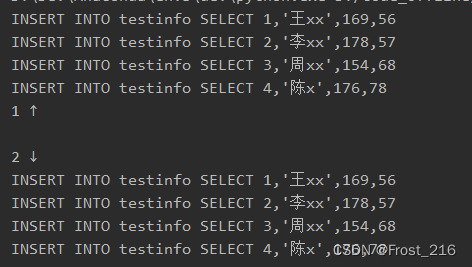``````CREATE TABLE testinfo(
id INT NOT NULL,
name VARCHAR(64) NULL,
height INT NULL,
weight INT NULL
)``````

``````conn = conn()
for i in range(len(df)):
id = df.iloc[i]
name = df.iloc[i]
height = df.iloc[i]
weight = df.iloc[i]

strsql = f"INSERT INTO testinfo SELECT {id},'{name}',{height},{weight}"
print(strsql)

cursor = conn.cursor()
cursor.execute(strsql)  # 执行sql语句
conn.commit()  # 提交
cursor.close()``````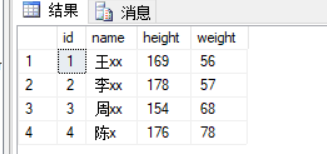### 导出：

1. 通过表名直接从数据库导出

2. 利用pandas的dataframe导出

#### 1. 通过表名导出excel：

``````import xlwt

def export_excel(table_name):
# 连接数据库，查询数据
host, user, passwd, db = '127.0.0.1', 'root', '123', 'bms'

cur = conn.cursor()
sql = 'select * from %s' % table_name
cur.execute(sql)  # 返回受影响的行数

fields = [field for field in cur.description]  # 获取所有字段名
all_data = cur.fetchall()  # 所有数据

# 写入excel
book = xlwt.Workbook()

for col, field in enumerate(fields):
sheet.write(0, col, field)

row = 1
for data in all_data:
for col, field in enumerate(data):
sheet.write(row, col, field)
row += 1
book.save("%s.xlsx" % table_name)

export_excel("testinfo")``````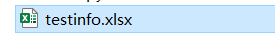#### 2. 利用pandas的dataframe导出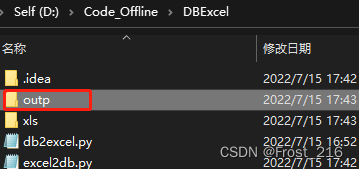``````strsql = 'select * from testinfo'

conn = conn()
cursor = conn.cursor()
cursor.execute(strsql)  # 执行sql语句
sqldata = cursor.fetchall() # 获取执行查询语句获得的结果数据
df = pd.DataFrame(data=sqldata) # 数据送入 dataframe
cursor.close()
print(df) # 查看 dataframe

# to_excel 导出
df.to_excel('outp/testinfo.xls', sheet_name='sheet1')``````